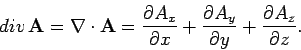Divergence of downhill flowing water

I just learned that an incompressible fluid must have zero divergence within a given control volume. Given that the divergence of a fluid at a point(x,y,z) can be found by taking the scalar sum of the of the x, y, z acceleration vectors at the given point, wouldn't this mean that water flowing downstream, consequently accelerating, would have a nonzero divergence? Does that mean that accelerating water is compressible?

sophiecentaur
Gold Member
If the water is in an open channel then it could be accelerating and the level of the water could change to allow the same volume flow past a given point and allow the water to be going faster.
In a pipe, the water wouldn't accelerate.

mfb
Mentor
No. The stream will simply get thinner.

You can test this yourself with a stream of tap water falling down. The stream gets thinner downwards.
If the stream gets too thin, it will break into individual drops.

•sophiecentaur
If the water is in an open channel then it could be accelerating and the level of the water could change to allow the same volume flow past a given point and allow the water to be going faster.
In a pipe, the water wouldn't accelerate.

I understand that the flux over a closed control volume would be zero due to the change in water level. I can't however seem to reconcile this with the definition of divergence being the scalar sum of the acceleration vectors at a given point. At any given point in an open channel, the water should have a nonzero divergence according to this definition.I am referring to this definition, where A is the velocity vector field of the fluid. In the case of downhill accelerating water, dAx/dx and dAy/dy should be nonzero. Therefore, div A should be nonzero.

Attachments

sophiecentaur
Gold Member
At any given point in an open channel, the water should have a nonzero divergence according to this definition.
No. Take an elemental cubic volume in the stream.The flux into the upstream face will be the same as the sum of the fluxes in and out of the other five faces. That is a description of the divergence.

sophiecentaur
Gold Member
View attachment 215559 I am referring to this definition, where A is the velocity vector field of the fluid. In the case of downhill accelerating water, dAx/dx and dAy/dy should be nonzero. Therefore, div A should be nonzero.
Why are you not considering the derivative in the z direction?

Assuming the water were flowing down a smooth hill the acceleration would only occur in two directions. Depending on the coordinate axis orientation chosen, it could be any two. In this case, I take the z derivative to be zero.

sophiecentaur
Gold Member
Assuming the water were flowing down a smooth hill the acceleration would only occur in two directions. Depending on the coordinate axis orientation chosen, it could be any two. In this case, I take the z derivative to be zero.
Not true. The stream is getting thinner. All three dimensions are involved.
You need to work towards the accepted solution rather than finding arguments to show it’s wrong. You have not found a ‘flaw’ in the theory.

Dale
Mentor
2021 Award
the definition of divergence being the scalar sum of the acceleration vectors at a given point.
You are mixing up spatial derivatives and the time derivative.

Chestermiller
Mentor
Each of the velocity derivatives in the divergence can be interpreted kinematically as the rate of linear strain in that direction. Just as in the case of thermal expansion, where the sum of the 3 linear strains is equal to the volumetric strain, here, the sum of the three rates of linear strain is equal to the rate of volumetric strain. But since the material is incompressible, its rate of volumetric strain is equal to zero.